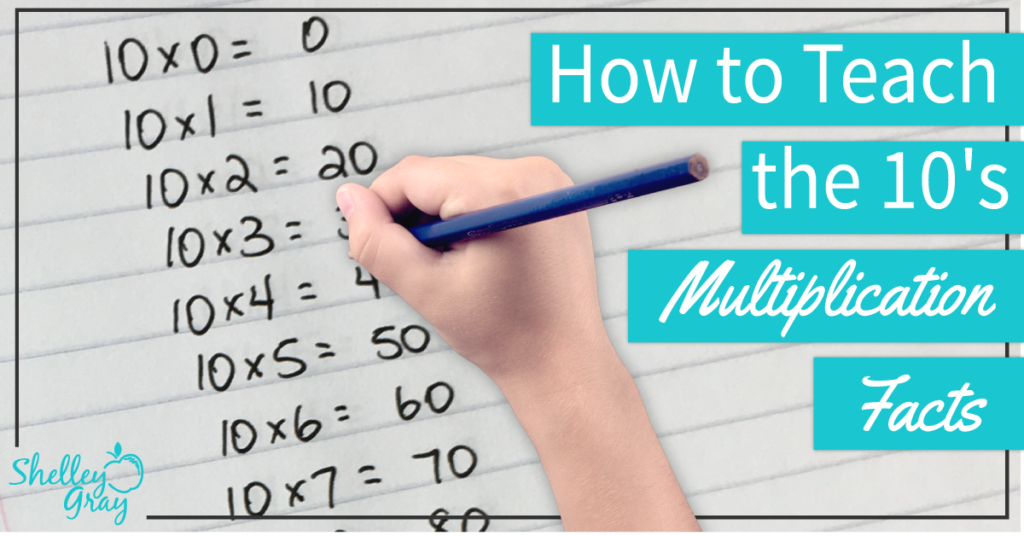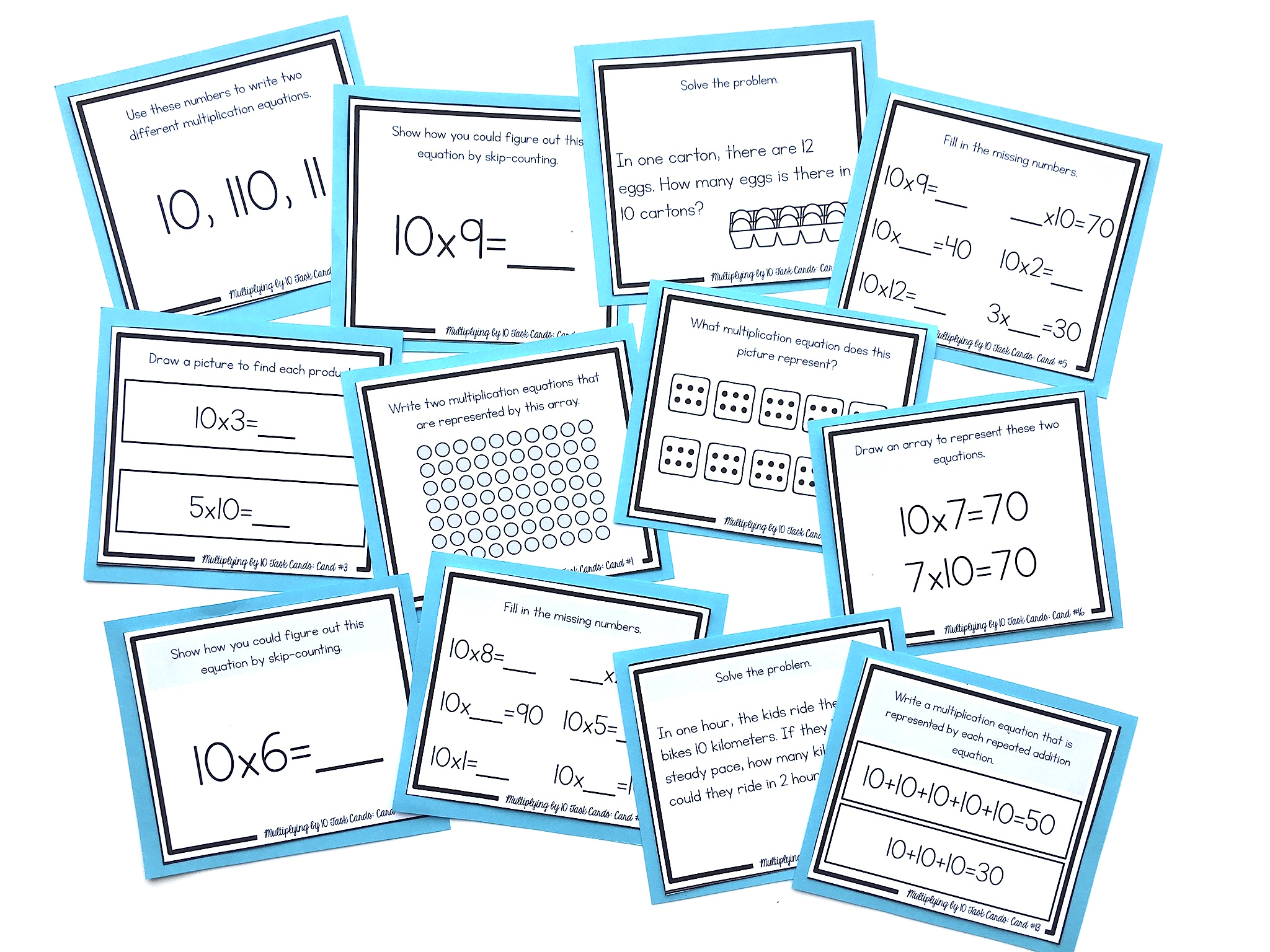# How to Teach the 10’s Multiplication FactsThe 10’s multiplication facts are typically an easy set of facts to learn.

When you teach to multiply by 10, be sure that your students understand the “number sense” behind multiplying by 10. Teach that when you multiply by 10, you simply shift the digits one place to the left. A zero is used as a place holder. For example, when you multiply 3×10, you shift the digits one place to the left and get a product of 30.

When you multiply 6×10, you shift the digits one place to the left to get the product of 60.

Here’s a video to illustrate this process:

Why not simply teach to add a 0?

Adding a 0 does work for whole numbers. But later on when they begin working with decimals, they will have to un-learn this trick. Think about a problem like 1.34 x 10. Do we simply add a 0 to make 1.340? NO! Instead, we shift all of the digits one place to the left to make 13.4. Learning this now will ensure that they do not have to un-learn later on.

Students will likely discover, on their own, that when you multiply a number by 10, you just add a 0. Be sure to reinforce that this only works with whole numbers.

It’s also very important to reinforce the commutative property.

This means that the order of factors does not change the product. For example, if students are faced with an expression like 7×10, they should see the 10 as one of the factors, and know that they can use the 10’s strategy to solve this equation.

NEXT STEPS

Reinforce the 10’s multiplication facts with this set of task cards. Students will learn conceptually through problem-solving, using arrays, strategic thinking, finding missing numbers, skip-counting, picture representations, and more:OR FIND THE FULL BUNDLE OF MULTIPLICATION TASK CARDS HERE.